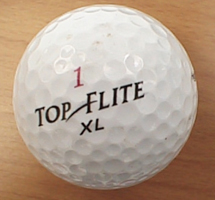### MS 095 PreAlgebra Spring 2005 Quiz 4 • Name:

1. __________ A golf ball rolls 28.65 meters in 8.61 seconds. How fast, in meters per second, was the golf ball traveling?
2. __________ A golf ball rolls 28.65 meters in 5.16 seconds. How fast, in meters per second, was the golf ball traveling?
3. __________ Which golf ball was moving faster, the golf ball in question one or the golf ball in question two?
4. __________ A golf ball travels at 4.0 meters per second for 7 seconds. How far did the golf ball travel?
5. __________ A golf ball travels at 7.0 meters per second for a distance of 35 meters. How long did it take the golf ball to travel that 35 meter distance?
6. __________ Toughie: Lee Ling can jog the nine kilometers from the college to his house in about an hour. A kilometer is one thousand meters. An hour is 60 minutes, and a minute is 60 seconds. How fast, in meters per second, was Lee Ling?
7. __________ Another toughie: A golf ball has a diameter of 4.267 cm. What is the volume of the golf ball?